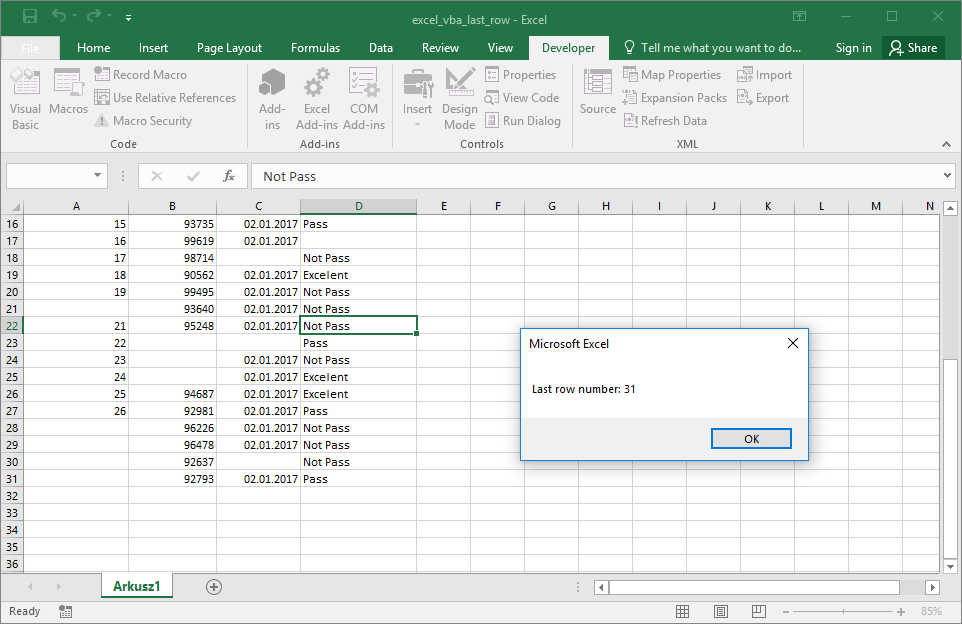# How to find last filled row in VBA Excel

### 1. Search for the last row number in the Excel table using VBA.

Often in VBA we need to add another row or data record into the Excel table. To do this, it is usually necessary to find the location of the last non-empty row. To find the last completed record in the sheet, we can use the simple VBA code:

The code listed above allows us to know the row number in column A. The row number is returned in the MsgBox window. The method is good as long as column A is always filled. For example, this is a column identifying record id. When some of cells are empty, or in your table are empty rows between records, you can use macro based on the find functionality in Excel. The code listed below also works in situations where there are entire empty columns or blank rows between the data. If you want to find using VBA last row index, just use this code:Excel VBA How To – Find VBA last row

Example is a modification of the Find / Change functionality macro in Excel. The main difference is that the expression we are looking for in the sheet is changed to the “*” value representing every possible expression.

### 2. Search for the last column number in the Excel table using VBA

If you also want to find using VBA last column index, you need to modify last example. You need to add short code refered to column address. An example is listed below.

```'officeinside.org
Sub FindLastRow()

Dim rngCell As Range
Set rngCell = Cells.Find(What:="*", After:=Cells(1, 1), LookIn:=xlFormulas, LookAt _
:=xlPart, SearchOrder:=xlByRows, SearchDirection:=xlPrevious, MatchCase:= _
False, SearchFormat:=False) '.Activate
If Not rngCell Is Nothing Then MsgBox "Last row number: " & rngCell.Row & " and last column number: " & rngCell.Column

End Sub```Excel VBA How To – VBA last row

In this way, you have found the index of the last row and column in the table using VBA. Excel file with the examples is attached below:

excel_vba_last_row.xlsm

If you are looking for similar examples of the practical programming in VBA, I invite you to visit this section: Excel VBA How To.